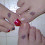## Monday, August 1, 2016

### #MTBoSBlaugust Algebra 2 Curriculum Overview

For the past few years our Algebra 2 curriculum has been focused on functions.  First we explore them graphically, then we apply them in problem solving.  Each year we tweak the sequence just a bit.  But here is a general overview of suggested units by months (not exact months).

August: Introduction to Functions

• 8 functions:  linear, absolute value, quadratic, cubic, square root, exponential, logarithmic, rational
• Function notation
• Transformations
• Domain/Range

September:  Systems of Equations

• Linear Systems, Nonlinear Systems, 3 variable systems
• Solving by Graphing, Substitution, Elimination, Gaussian, Matrices
• Linear Inequalities and Optimization Problems
• Application Problems

• Attributes of the Quadratic Function, Analysis of the Graph
• Transformations
• Introduction of parabola as a conic section (conics taught in PreCalc)
• Forms of the equation: standard form, vertex form, transformation form, conics form
• Writing the equation that fits given criteria
• Solving Quadratics: factoring, roots, completing the square, formula
• Complex Numbers
• Application Problems

November:  Cubic Functions

• Attributes of the Cubic Function, Analysis of the Graph
• Transformations
• Factoring cubic patterns
• Solving by graphing or factoring

December: Polynomials

• Basic Operations including long division, synthetic division
• Factoring
• Polynomial Theorems - roots, factors,
• Key attributes of graphs - end behavior, number of roots
• Regression Equations - best fit polynomial models
January: Rational Functions
• Key attributes and analysis of graph
• Transformations
• Holes, Asymptotes (not slant - precalc)
• Basic Operations with Rational Expressions
• Simplify Complex Fractions
• Solve Rational Equations
• Application Problems
February: Concept of Inverse Functions and Radical Functions
• Inverse Functions
• Solving Problems using inverses
• Key Attributes of the square root and cube root functions
• Transformations
• Rational Exponents
• Application Problems
March:  Exponential & Logarithmic Functions
• Attributes of exponential and log graphs
• Transformations
• Analysis of exponential function (growth vs decay)
• Writing an exponential function in recursive form
• Log Properties
• Finding the inverse
• Solving equations
• Application Problems
• Analyzing Linear, Quadratic or Exponential
April:  Absolute Value Functions
• Introduction to Piecewise Functions
• Key attributes of the graph
• Transformations
• Solving equations and inequalities
• Application Problems
May:  Other Topics ... select as needed
• Wrap up previous topics  (most likely scenario)
• Review functions - finding commonalities and differences
• Introduce Sequences/Series (taught in PreCalc)

1.How much do you go into conics? At my school, conics are all done in one mini-unit (we only do parabolas and circles). For parabolas we write the equation after being given any two of the three: vertex, directrix, or focus, and then graph. We also convert from standard form to vertex form by completing the square, and we do the same for circles (except that's more of double completing the square). We also find the equation of a circle given endpoints of a diameter.

1.Our district separated conics over years. Circles are addressed in Geometry; Parabolas in Algebra 2; and the rest in PreCal. Our PreCal teachers do all four conic sections.

For parabolas we do the same as you ... vertex, directrix, and focus. This past year we combined the conics features (directrix and focus) in our unit on quadratics.

2.Can I just say again how much I'm going to miss having you in the classroom? Thank you for sharing so many awesome ideas over the years!

1.Thank you, dear one! I'll keep sharing until what I have gets old!

I'm excited to help with your Algebra 2 ... if there is anything at all I can do!

3.I will be teaching Algebra II which I have not taught since 2012. Are there sites that you have found to be more beneficial for me. I am familiar with Kuta and Desmos. We were using PMI. Any info is appreciated.

1.Start here: https://ispeakmath.org/2016/07/23/calling-all-algebra-2-teachers-mtbos-alg2chat/

Add yourself to the list. Also note all the Alg 2 bloggers.

What curriculum will you use this year?

4.What does your first topic "8 functions: linear, absolute value, quadratic, cubic, square root, exponential, logarithmic, rational" look like? I see that you go into more detail of these functions later in the year.

1.We introduce the parent functions only. Students create a booklet about the attributes of the parent functions ... a graph, the domain, range, key points, asymptotes, etc. Students practice domain and range - written as inequalities, set notation, and interval notation. They also practice finding the value of a function f(x), f(4), etc. We introduce transformations by moving a "monster" ... moving point by point.

After that unit we go through each function with much more depth.